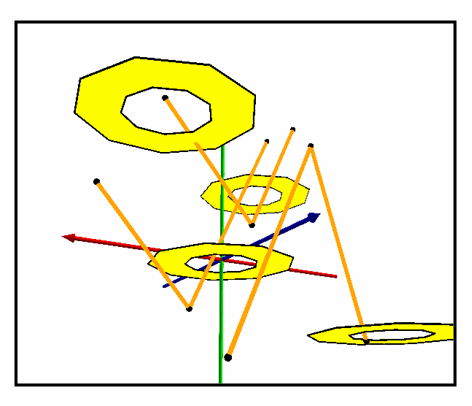This document is archived and information here might be outdated.  Recommended version.

IRelationalOperator3D.Disjoint3D Method (ArcObjects .NET 10.7 SDK)
 ArcObjects Help for .NET developers > ArcObjects Help for .NET developers > ArcObjects namespaces > Geometry > ESRI.ArcGIS.Geometry > Interfaces > IR > IRelationalOperator3D Interface > IRelationalOperator3D.Disjoint3D Method
 ArcGIS Developer Help

# IRelationalOperator3D.Disjoint3D Method

Indicates if the two geometries share no points in common. Negate this result to compute the Intersect relation.

```[Visual Basic .NET]
Public Function Disjoint3D ( _
ByVal pOther As IGeometry _
) As Boolean
```
```[C#]
public bool Disjoint3D (
IGeometry pOther
);
```
```[C++]
HRESULT Disjoint3D(
IGeometry* pOther
);
```
```[C++]
Parameters
pOther
pOther is a parameter of type IGeometry*
```

#### Product Availability

Available with ArcGIS Engine, ArcGIS Desktop, and ArcGIS Server.

#### Description

Determines whether two z aware geometries intersect in 3D space.  Two geometries are disjoint if their intersection is empty.  Two geometries intersect if disjoint is FALSE.

[C#]public static void TestIntersection()

{

IGeometry polylineGeometry = GetPolylineGeometry();

IGeometry polygonGeometry = GetPolygonGeometry();

IRelationalOperator3D
relationalOperator3D = polylineGeometry as IRelationalOperator3D;

bool intersect = !(relationalOperator3D.Disjoint3D(polygonGeometry));

//intersect = true

}